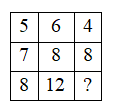Find the missing character (?) in the figure given below.

# Find the missing character $\left(?\right)$ in the figure given below.1. A

16

2. B

12

3. C

5

4. D

6

Fill Out the Form for Expert Academic Guidance!l

+91

Live ClassesBooksTest SeriesSelf Learning

Verify OTP Code (required)

### Solution:

For any column, take the product of the numbers in row 1 and row 2 and then add the digits of the results obtained to arrive at the number in row 3 of that column.

$\begin{array}{l}5×7=35\to 3+5=8\\ 6×8=48\to 4+8=12\\ 4×8=32\to 3+2=5\end{array}$+91

Live ClassesBooksTest SeriesSelf Learning

Verify OTP Code (required)